# Logic Operation OR

## OR Gate

In its most basic configuration, the gate has two inputs and one output. The output is one when one input is one or when both inputs are one as shown below.

 x1 x2 z 0 0 0 0 1 1 1 0 1 1 1 1

 Tip In some problems, there is just one data set to perform the training and the validation. In these cases, the given data set must be divided to create the training set and the validation set. Typically this process is randomly performed.

 Problem 1 Create a New Project called MappingOR to build an appropriate set for the OR gate. Use 128 training cases. In this case, we will not need the BuildTrainSet.lab file nor the BuildValidSet.lab as shown.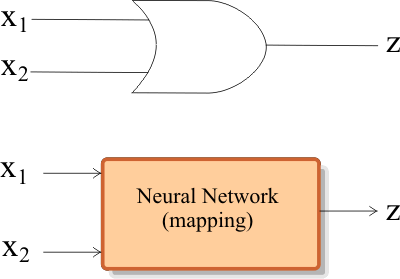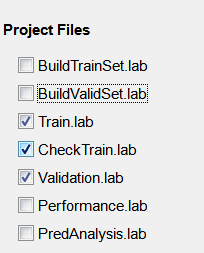Solution 1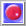Neural Lab open Neural Lab.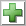Add File click the button to add the BuildDataSet.lab file. Write the code show below.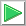Run click the button to execute the code. If you do not have any errors, the data set will be generated and displayed on the variable list and the file list. Click on the file to see its contents.

 MappingOr\BuildDataSet.lab int numCases = 128; Matrix input; input.CreateRandom(numCases, 2, 0.0, 2.0); // Random values in [0 2) input= floor(input); // Round any value in [0 1) to 0 and any value in [1 2) to 1 Matrix dataSetTarget; dataSetTarget.Create(numCases, 1); //_________________________________________ Compute the target for each case int row = 0; for(row =0; row < numCases; row++) {      if (input[row] == 1 || input[row] == 1)      {           dataSetTarget[row] = 1;      }      else      {           dataSetTarget[row] = 0;      } } //_________________________________________ Create some noise Matrix noise; noise.CreateRandom(numCases, 2, 0.0, 1.0); // Random values in [0 1) //_________________________________________ Contaminate the input with noise Matrix dataSetInput = 0.9 * input+ 0.1 * noise; //_________________________________________ Save the data set dataSetInput.Save(); dataSetTarget.Save();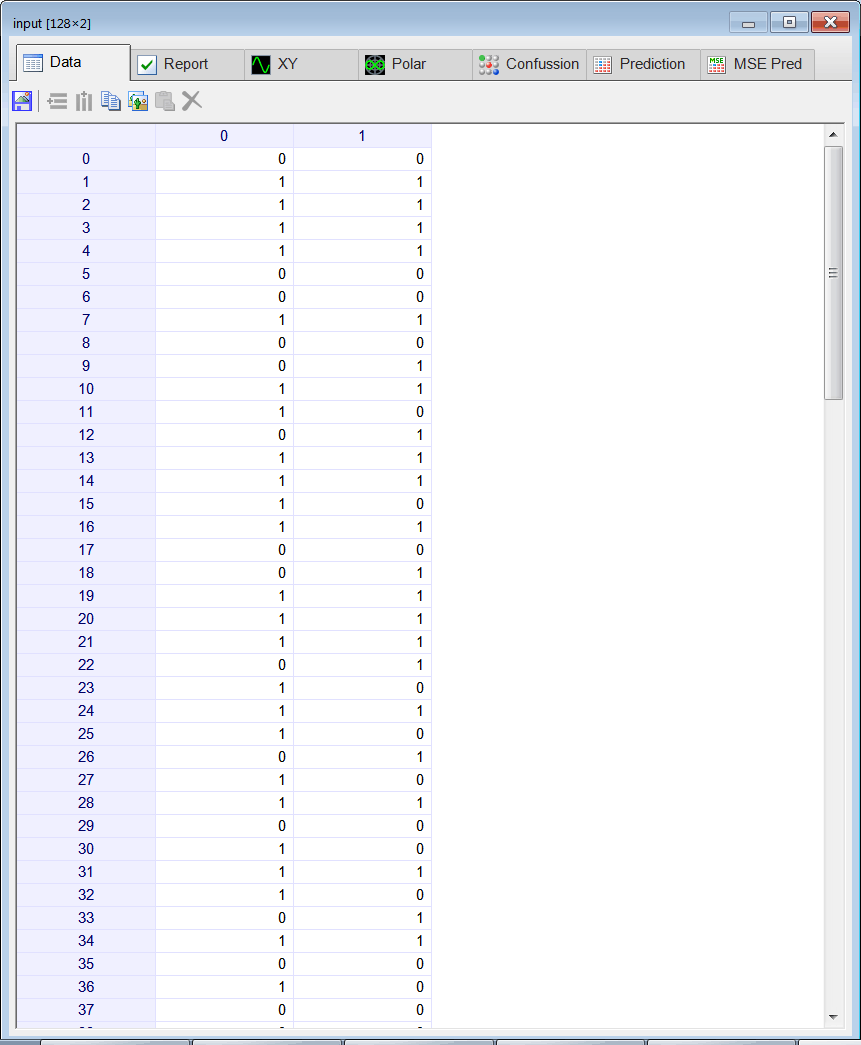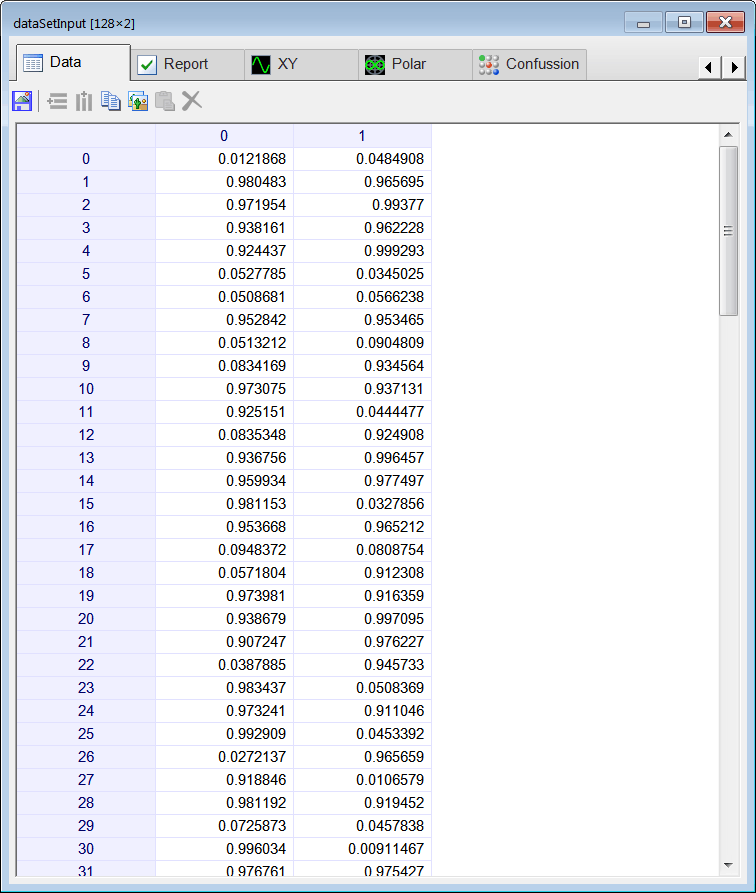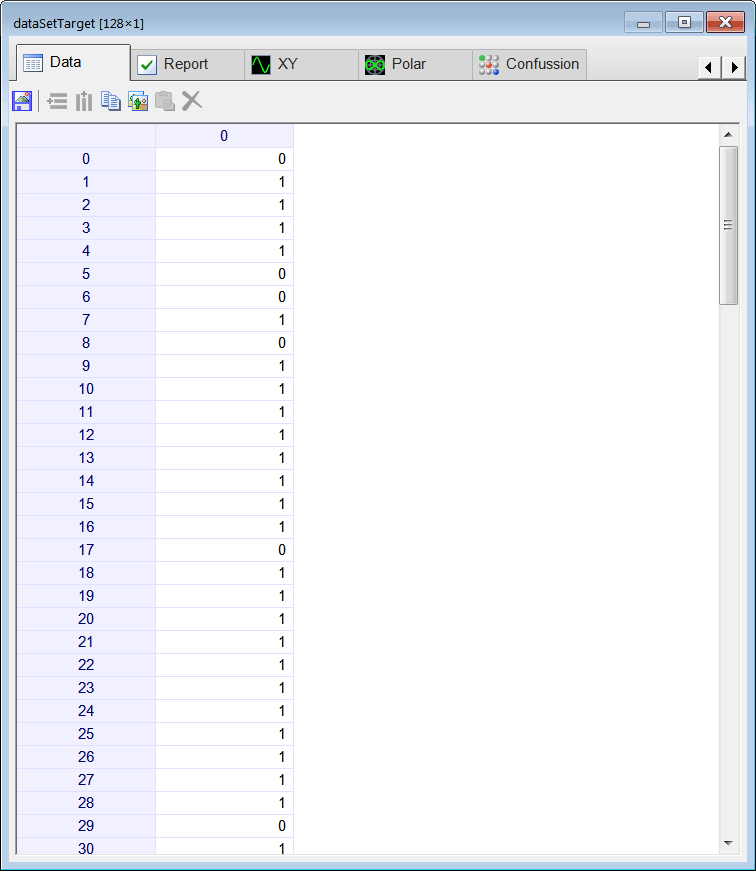Problem 2 Add the Split.lab file to build the training set and the validation set from the data set. Use 80 % of the cases for training, and 20 % of the cases for validation, see figure below.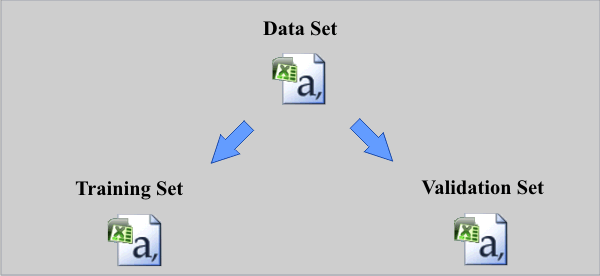Solution 2 After editing the file,Run click the button to execute the code. If you do not have any errors, the training set and the validation set will be generated and displayed on the variable list and the file list.

 MappingOr\Split.lab //______________________________________ Load the data set Matrix dataSetInput; dataSetInput.Load(); Matrix dataSetTarget; dataSetTarget.Load(); int totalCases = dataSetInput.GetRowCount(); int trainCount = toint(0.8 * totalCases); //The 80% of the cases will be used for training //_______________________________________ Generate randomly the case indexes for training Vector trainCases; trainCases.CreateRandomSet(trainCount , totalCases-1); //_______________________________________ Build the training set Matrix trainSetInput= dataSetInput.GetRows(trainCases); Matrix trainSetTarget= dataSetTarget.GetRows(trainCases); //_______________________________________ Save the training set trainSetInput.Save(); trainSetTarget.Save(); //_______________________________________ Build the validation set Matrix validSetInput = dataSetInput; validSetInput.DeleteRows(trainCases); // Matrix validSetTarget = dataSetTarget; validSetTarget.DeleteRows(trainCases); //_______________________________________ Save the validation set validSetInput.Save(); validSetTarget.Save();Problem 3 Edit the Train.lab file to design and train an ANN for the OR gate. Use one hidden layer and one neuron in this layer. Train the ANN using a genetic algorithm as shown:      Initial Population Size = 100      Number of Generations = 100      Over Population = 1.5      Mutation Probability = 0.001      Crossover Probability = 0.8      Goal (desired mse) = 0.00001 A genetic algorithm is an optimization method inspired on biological process that in this specific case is used for ANN training.

 Problem 4 Edit the CheckTraining.lab file to check the training: (a) Compute the mean squared error for the ANN using the training set. (b) Plot the error for each training case. (c) Save the plot as a vector image (checkTraining.emf and checkTraining.pdf)

 Problem 5 Edit the Validation.lab file to perform the validation of the ANN. (a) Compute the mean squared error for the ANN using the validation set. (b) Plot the error for each validation case. (c) Save the plot a as a vector image (validation.emf and validation.pdf)

 Problem 6 Generate a report in Microsoft Word. Write some conclusions in the report focusing on the problems that were faced during the simulation and how these problems were or could be solved.

 Problem 7 Discuss the code in problem 2.